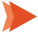Kick Start Your Accounting Journey, We Help You to Start from Scratch

# High Low Method in Accounting Definition, Examples, Pros, and Cons

The high low method in accounting is a standard method for analyzing what percentage of a cost is fixed and variable.

Using this method, the accountant determines the maximum and lowest output levels during a set period of time and the overall manufacturing costs connected with these levels.

The lowest number of units manufactured is then subtracted from the greatest number of units manufactured.

The lowest output level’s total cost of manufacturing is then subtracted from creating the most significant number of units. The variable costs per unit can be calculated by dividing the cost difference by the change in output levels.

To put it another way, management uses the high low method accounting formula to divide and chart the fixed and variable costs connected with producing a good.

To illustrate the average, a line is created linking the lowest and highest values.

## High Low Method Accounting Formula

The high low method accounting is a straightforward way to divide fixed and variable costs using a few formula steps. You must first evaluate the variable and fixed cost components before entering the values into the cost model formula.

(Highest activity cost Lowest activity cost) (Highest activity units Lowest activity units) = variable cost per unit

You can determine the fixed cost after you have the variable cost per unit.

Fixed cost = Cost of the most expensive activity (Variable cost per unit x Highest activity units)

or

Fixed cost – Lowest activity cost (Variable cost per unit x Lowest activity units)

Then, using this formula, calculate the high–low cost using all of the results:

Fixed cost + (Variable cost + Unit activity) = High-low cost

## High Low Method Example

Let’s look at an example to help you understand how to calculate with this method.

The table below depicts a company’s overall cost for various production levels in the year’s first six months.

 Units Total Cost 3000 \$59,000 2720 \$55,000 2500 \$52,500 2150 \$50,500 1950 \$47,500 1250 \$38,000

To figure out the variable and fixed costs, use the formula above to figure out the variable cost per unit. Here, x2 equals 3000 and y2 equals \$59,000, while x1 equals 1250 and y1 equals \$38,000.

Using the calculation (\$59,000 \$38,000) (3,000 1,250), we arrive at a variable cost of \$12 per unit.

Let’s figure out the fixed cost. Adding the value to the equation:

The fixed price will be \$59,000 (\$12,300) or \$38,000 (\$12,250). As a result, the total fixed cost is \$23,000.

We can construct the cost volume function now that we have both fixed and variable costs. y = \$23,000 + 12x will be the answer.

## What Are the Benefits of Using the High Low Method of Accounting?

The benefits of high low method accounting are below:

• The lack of formality required by the high-low technique is one of its advantages. These figures can be analyzed by the accountant utilizing data from monthly expenses and activity levels. He doesn’t have to talk to anyone outside the organization to figure out the fixed costs or the variable rate per unit.
• The computation does not necessitate the use of sophisticated instruments or programs.
• This strategy is simple to apply and comprehend. This approach only requires two data values to generate the cost behavior.
• This strategy also makes it simple for analysts and accountants to determine future unit costs.
• Another advantage of this method is that the fixed and variable costs may be calculated using only two sets of numbers. These factors include the level of exercise and the total expenditure. To calculate the entire cost, the accountant examines the account’s financial transactions across several months.

## What Are the Disadvantages of the High-Low Accounting Method?

The disadvantages of high low accounting are below:

• Because this method ignores step cost, it may produce erroneous results. Step costs are the expenses incurred by a company at a given volume. If the cost falls somewhere between the high and low values, the high-low approach may produce erroneous results.
• First drawback of the high-low technique is that the results are estimations rather than precise figures. A vendor should be contacted directly by an accountant who needs to know the exact dollar amount of fixed expenses each month.
• The high low technique completely ignores inflation. When inflation is factored in, the variable and fixed costs will differ. We’ll have to alter it to fit our calculations; otherwise, the figure won’t tell the complete story.
• Another flaw with this method is that the fixed and variable costs are calculated using only two sets of numbers. These factors include the level of exercise and the total expenditure. To calculate the entire cost, the accountant examines the account’s financial transactions across several months.
• This strategy implies that the cost and activity have a linear relationship. It is, on the other hand, the oversimplification of cost behavior. We can use ABC to better picture the cost and volume behavior (Activity-based costing).

#### Final Words

By displaying all transactions and connecting the lowest and highest points, the high-low method determines the average cost of purchasing a good or generating a product in a graphical manner.

However, the two extreme data points do not provide exceptionally reliable results, as previously stated.

As a result, you should not rely only on this information to determine the true variable and fixed costs.

It’s helpful if you need a rapid estimate of variable and fixed expenditures. However, don’t rely on it for accurate findings, as semi-variable costs play an important part and can be significant at times.